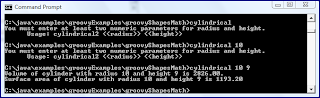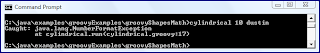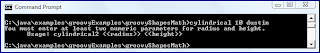## Saturday, November 21, 2009

### Using Groovy to Check Seventh Grade Homework

Using Groovy to Calculate Volume and Surface Area of a Cylinder

I have recently been involved in checking a seventh grader's math homework to make sure it was done correctly and to help her learn the new concepts. The recent focus of her class has been on volumes and surface areas of various geometric shapes. These can be tedious to check the arithmetic on. Although I do have a HP48GX calculator (my HP48SX got stolen about 3/4 of the way through my degree) from the days of my undergraduate degree in electrical engineering, its built-in formulas use a definition of pi with many more significant digits than the seventh graders use (3.14). There are other options available (such as Google search's online calculator function), but the potentially different precision of PI is still an issue.

For a variety of reasons (inner geekiness, long-term laziness, and because I can use it as a blog topic) I decided to write a small application to make these checks easier. I did not want to directly deal with floating-point arithmetic issues and Java's handy BigDecimal came to mind. At about the same time, I realized that this was a perfect use for Groovy: I wanted a Java language feature but wanted to be able to write concise, script code.

The volume (`V = Πr2h`) and surface area (`SA = 2Πr2 + 2Πrh`) of a cylinder are the main topics for the current homework assignment. The Groovy code below calculates the volume and surface area of a cylinder given a radius as the first parameter and a height as the second parameter. It is assumed that the radius and height are in the same (unspecified) units.

`// cylindrical1.groovy//// Calculates geometric characteristics of a cylinder with given radius and height.//// Don't want to use Math.PI because want same accuracy as used in homeworkpi = 3.14radius = new BigDecimal(args)height = new BigDecimal(args)volume = pi * radius * radius * heightsurfaceArea = 2 * pi * radius * radius + pi * 2 * radius * heightprintln "Volume of cylinder with radius \${radius} and height \${height} is \${volume}."println "Surface area of cylinder with radius \${radius} and height \${height} is \${surfaceArea}"`

Running the script on several different values for radius and height of different cylinders is shown in the next screen snapshot.The script above does the job, but it can be improved even more. In the second version of this script, I add a conditional check that prints out usage information if the caller does not provide two numeric values for radius and height. I also use Groovy's GDK-extended String method toBigDecimal() rather than the more traditional approach of passing the String to the BigDecimal constructor.

`// cylindrical2.groovy//// Calculates geometric characteristics of a cylinder with given radius and height.//if (args.length < 2){   println """You must enter at least two numeric parameters for radius and height.      Usage: cylindrical2 <<radius>> <<height>>"""   System.exit(args.length)}// Don't want to use Math.PI because want same accuracy as used in homeworkpi = 3.14radius = args.toBigDecimal()height = args.toBigDecimal()volume = pi * radius * radius * heightsurfaceArea = 2 * pi * radius * radius + pi * 2 * radius * heightprintln "Volume of cylinder with radius \${radius} and height \${height} is \${volume}."println "Surface area of cylinder with radius \${radius} and height \${height} is \${surfaceArea}"`

The "happy path" output for this version of the script is the same as for the first version and will not be shown here. However, the usage information provided when an insufficient number of parameters is provided is shown in the next screen snapshot.One problem still remaining with this script is that non-numeric values passed in for radius or height can be problematic as demonstrated in the next screen snapshot in which the second command-line argument (expected height) is a String.One approach for dealing with this is to use the GDK-extended String method isNumber(). This is the approach applied in the next Groovy code listing.

`// cylindrical3.groovy//// Calculates geometric characteristics of a cylinder with given radius and height.//if (args.length < 2 || !args.isNumber() || !args.isNumber()){   println """You must enter at least two numeric parameters for radius and height.      Usage: cylindrical2 <<radius>> <<height>>"""   System.exit(args.length)}// Don't want to use Math.PI because want same accuracy as used in homeworkpi = 3.14radius = args.toBigDecimal()height = args.toBigDecimal()volume = pi * radius * radius * heightsurfaceArea = 2 * pi * radius * radius + pi * 2 * radius * heightprintln "Volume of cylinder with radius \${radius} and height \${height} is \${volume}."println "Surface area of cylinder with radius \${radius} and height \${height} is \${surfaceArea}"`

The improved output now resulting from the providing of a non-numeric value for height is shown in the following screen snapshot.Although this basic command-line argument checking is not difficult, it turns out that Groovy has more sophisticated command-line argument support built in. Groovy takes advantage of the Apache CLI library that I have previously blogged on and makes it even easier to use via `CliBuilder`. The next code listing demonstrates Groovy code using `CliBuilder` to handle command-line arguments. In addition, it provides several other examples of additional "grooviness."

`#!/usr/bin/env groovy// cylindrical4.groovy//// Calculates geometric characteristics of a cylinder with given radius and height.//def cli = new CliBuilder(usage: 'cylindrical -r radius_arg -l height_arg [-u arg]')cli.with{   h(longOpt: 'help', 'Usage Information')   r(longOpt: 'radius', required: true, type: Number, 'Radius of Cylinder', args: 1)   l(longOpt: 'length (height)', required: true, type: Number, 'Height of Cylinder', args: 1)   u(longOpt: 'units', 'Units of Supplied Radius and Height', args: 1)   p(longOpt: 'pi', 'Value of pi used in calculations', type: Number, args: 1)}def opt = cli.parse(args)if (!opt || opt.h) return   // parsing error occurred or usage was requestedif (!opt.r.isNumber()) {println "Radius must be numeric."; cli.usage(); return}if (!opt.l.isNumber()) {println "Height must be numeric."; cli.usage(); return}def radius = opt.r.toBigDecimal()def height = opt.l.toBigDecimal()def printableUnits = opt.u ?: "units"    // "Elvis" operatordef pi = opt.p ? opt.p.toBigDecimal() : Math.PI// Don't want to use Math.PI because want same accuracy as used in homework//def pi = 3.14def volume = pi * radius * radius * heightdef surfaceArea = 2 * pi * radius * radius + pi * 2 * radius * heightprintln "All calculation are using \${pi} as the value of 'pi'"println "Volume of cylinder with radius \${radius} \${printableUnits} and height \${height} \${printableUnits} is \${volume}."println "Surface area of cylinder with radius \${radius} \${printableUnits} and height \${height} \${printableUnits} is \${surfaceArea}"`

The use of Groovy's built-in support for command-line argument via Apache CLI and `CliBuilder` facilitates easy development of command line options with short (single hyphen) and long (double hyphen) names. Usage information is readily available via `CliBuilder` as well. In the code above, I used Groovy's with() to group the calls on my "cli" object.

Because CliBuilder is so easy to use, I threw in two more options to make the script output potentially more interesting and flexible. I also have support for radius and height to be more specifically specified so there is less change of switching the two numeric values on accident. The new command-line options allow the user to optionally specify the units of measurement and the value of PI used. If the units are not specified, the string "units" is used (thanks to the Elvis operator) and if the value of PI is not specified, the value from Math.PI is used by default (thanks to traditional Java's ternary operator).

The next screen snapshot demonstrates the flexibility of this final version of the script for calculating cylindrical volume and surface area.As the screen snapshot above demonstrates, `CliBuilder` has allowed me to easily apply great flexibility and usefulness in the my script. Other online posts on Groovy and CliBuilder include Groovy CLIBuilder in Practice, Groovy Command Line Options, Groovy CliBuilder with Multiple Arguments, and Groovy Script: Get SSL Cipher and CLIBuilder Example.

Observations

Groovy Uses of Standard Java APIs and Third-Party Java APIs and Libraries

One of the compelling advantages of Groovy is readily usable Java libraries and APIs and Groovy's built-in use of Apache CLI is a great example of this. For example, if the built-in, Apache CLI-powered command line arguments handling is not exactly what you want, you could consider using another Java-based library such as Args4j. For a direct Groovy alternative to the built-in support using Apache CLI, consider Groovy Option Parser. Java's `BigDecimal` (which Groovy uses underneath the covers for floating point types by default) was also particularly useful in this example.

Groovy is Great for Scripting

Groovy brings the advantages of Java's rich feature set while at the same time allowing the scripts to be written more script-like.

Programmer Laziness is a Different Type of Laziness

As many blog posts have already pointed out, the "lazy" programmer is often not "lazy" in the traditional sense of the word. The "lazy" programmer tends to go out of his or her way to reduce the tedious tasks that he or she must perform. He or she may be willing to expend significant effort to generate a script to avoid tedium that might, especially in an isolated case, take less time than writing the script. However, if the situation is one that is repeated, the generated script should earn back its time spent many times over.

Conclusion

Groovy is the perfect fit for writing a simple script to perform floating-point arithmetic with precise desired precision. I actually used the first version of the script in my case because it was done in about five minutes, but it was fun to make it more elegant and present that here. Another observation from all of this might be developers' strange sense of "fun." Groovy gives me a scripting language with the language richness and features of Java.